# How to Name an Angle in Four Ways

1. Math Lessons >
2. Naming Angles

It's time we closely examined an angle.

No matter how many angles are there in a diagram, each angle has its own unique name. We use the symbol '' followed by the name to represent an angle. The below links have more on how you can label an angle and in how many different ways you can accomplish this task.

Label an angle using the vertex and a point from each arm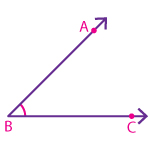ABC or CBA

Note:

The vertex should go between the two points.

Label an angle using only the vertex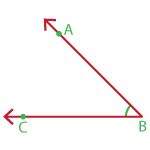B

Note:

When two or more angles share the same vertex, we don't use only the vertex to name the angles.

Label an angle using numbers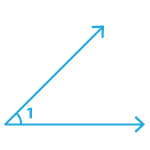1

Note:

Certain lower-case letters of the English alphabet like a, b, and c, and Greek letters like alpha (α), beta (β), gamma (γ), and theta (θ) are also used to represent angles.

Solved Examples

1. Name this angle.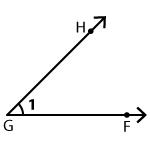There are four ways we can name this angle: FGH,HGF,G,1.

2. Locate DBC in the figure.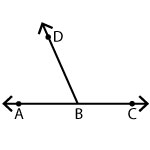DBC is highlighted in the image below.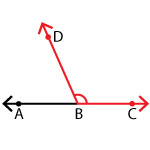Take up the challenge!

The below picture has V as its vertex. The task is for you to identify and name all the angles that can be formed from within the image.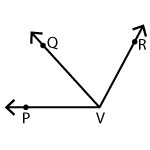PVQ or QVP, QVR or RVQ, and PVR or RVP